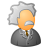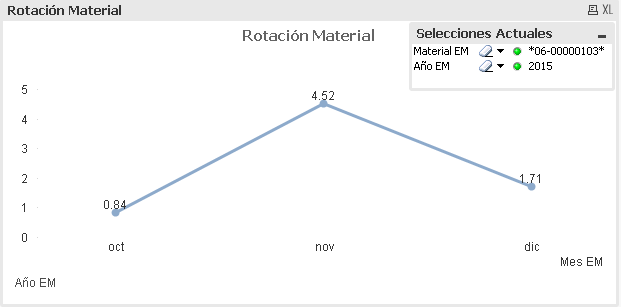# QlikView App Dev

Discussion Board for collaboration related to QlikView App Development.

cancel
Showing results for
Did you mean:Contributor III

## Differences Rangesum Above

Hi guys,

I have a query, I have 2 qvw files that have the same formula (cumulative sums) and but in one it comes out correctly, and in the other it comes out differently I have searched the root of the problem even ordering my table for the accumulated sum But still goes wrong. I hope you can help me.

Thank you so much.

Correct graphic:Incorrect graphic:1 Solution

Accepted SolutionsMVP

I think the problem here is the sort order of Fecha EM... If you have QV12, you can use this

=fabs(sum({<[MSEG.Clase de movimiento_BWART]={'201','221','261'}>} num(MSEG.Cantidad_MENGE)))

/

avg(

aggr(

rangesum( above( sum({\$<[Año EM],[Mes EM]>} num(MSEG.Cantidad_MENGE)),0,RowNo())),

[Material EM],([Fecha EM], (NUMERIC))

)

)

or you will have to fix the load order in the script to make this work

2 RepliesMVP

I think the problem here is the sort order of Fecha EM... If you have QV12, you can use this

=fabs(sum({<[MSEG.Clase de movimiento_BWART]={'201','221','261'}>} num(MSEG.Cantidad_MENGE)))

/

avg(

aggr(

rangesum( above( sum({\$<[Año EM],[Mes EM]>} num(MSEG.Cantidad_MENGE)),0,RowNo())),

[Material EM],([Fecha EM], (NUMERIC))

)

)

or you will have to fix the load order in the script to make this workContributor III
Author

Thank you so much!!